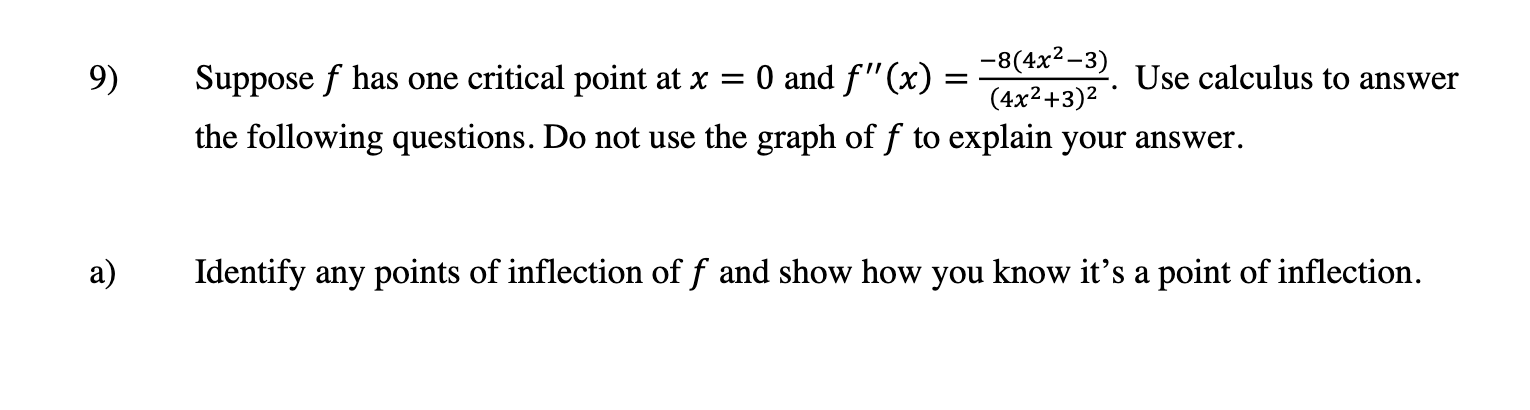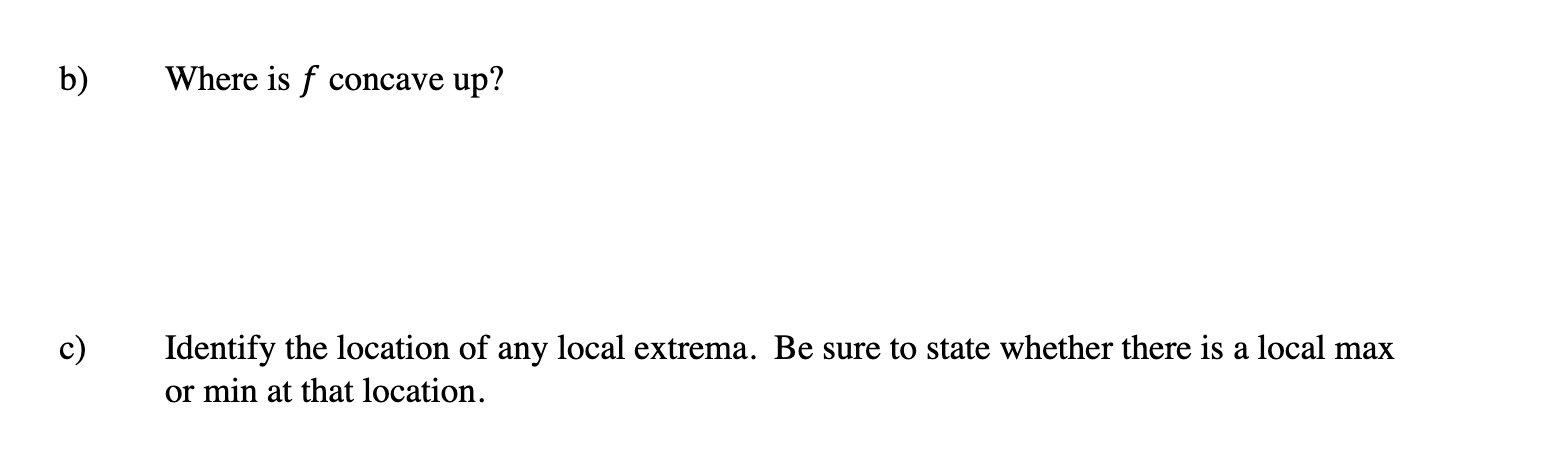### Create an Account

Home / Questions / 9) -8(4x2-3) Suppose f has one critical point at x = 0 and F"(x) = Use calculus to answer ...

# 9) -8(4x2-3) Suppose f has one critical point at x = 0 and F"(x) = Use calculus to answer (4x2+3)2 the following questions. Do not use the graph of f to explain your answer. a) Identify any points of

9) -8(4x2-3) Suppose f has one critical point at x = 0 and F"(x) = Use calculus to answer (4x2+3)2 the following questions. Do not use the graph of f to explain your answer. a) Identify any points of inflection of f and show how you know it's a point of inflection.
b) Where is f concave up? c) Identify the location of any local extrema. Be sure to state whether there is a local max or min at that location.Jun 02 2021 View more View LessSubscribe To Get Solution